# Horizontal Range Formula

Horizontal Range Formula

A projectile is an object that is given an initial velocity, and is acted on by gravity. The horizontal range of a projectile is the distance along the horizontal plane it would travel, before reaching the same vertical position as it started from. The horizontal range depends on the initial velocity v0, the launch angle θ, and the acceleration due to gravity. The unit of horizontal range is meters (m).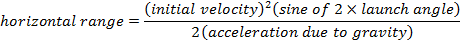R = horizontal range (m)

v0 = initial velocity (m/s)

g = acceleration due to gravity (9.80 m/s2)

θ = angle of the initial velocity from the horizontal plane (radians or degrees)

Horizontal Range Formula Questions:

1) A motorcyclist has set up a stunt with a ramp at the edge of a gorge 75.0 m wide. The ramp is inclined at 53.1° from the horizontal plane. She plans to take off from the ramp at a velocity of 28.0 m/s. At that velocity, what will be her horizontal range, and will she make it to the other side of the gorge?

Answer: The motorcyclist's horizontal range can be found using the formula: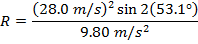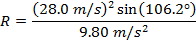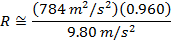The motorcyclist's horizontal range will be 76.8 m, if she takes off from the ramp at 28.0 m/s. This is slightly greater than the 75.0 m width of the gorge, so she will make it to the other side.

2) A pirate fired one of the ship's cannons to test its range. The cannon was set at an angle of 18.5°. The pirate watched the cannon ball, and noted that it hit the water 800 m away. What was the cannon ball's velocity when it left the cannon?

Answer: The velocity of the cannon ball can be found by rearranging the horizontal range formula: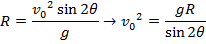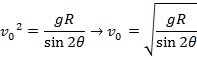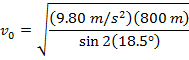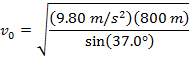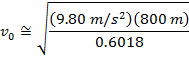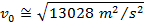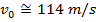The initial velocity of the cannon ball is approximately 114 m/s.